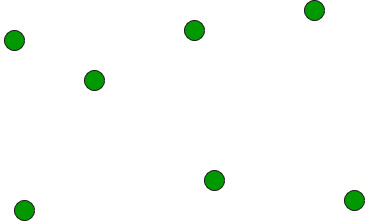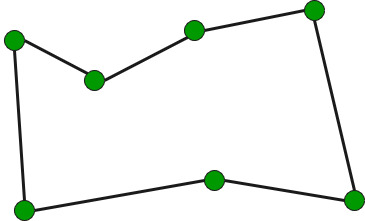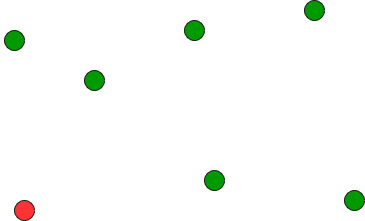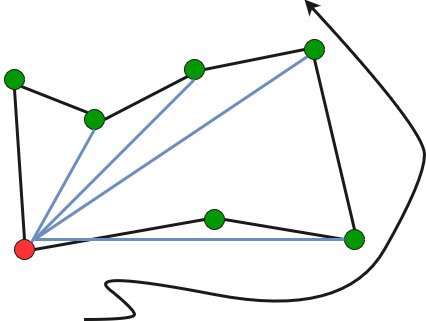# Find Simple Closed Path for a given set of points

Given a set of points, connect the dots without crossing.

``

Example:

```Input: points[] = {(0, 3), (1, 1), (2, 2), (4, 4),
(0, 0), (1, 2), (3, 1}, {3, 3}};

Output: Connecting points in following order would
not cause any crossing
{(0, 0), (3, 1), (1, 1), (2, 2), (3, 3),
(4, 4), (1, 2), (0, 3)}
```

We strongly recommend you to minimize your browser and try this yourself first.

The idea is to use sorting.

1. Find the bottom-most point by comparing y coordinate of all points. If there are two points with same y value, then the point with smaller x coordinate value is considered. Put the bottom-most point at first position.2. Consider the remaining n-1 points and sort them by polor angle in counterclockwise order around points. If polor angle of two points is same, then put the nearest point first.
3. Traversing the sorted array (sorted in increasing order of angle) yields simple closed path.How to compute angles?
One solution is to use trigonometric functions.
Observation: We don’t care about the actual values of the angles. We just want to sort by angle.
Idea: Use the orientation to compare angles without actually computing them!

Below is C++ implementation of above idea.

 `// A C++ program to find simple closed path for n points ` `// for explanation of orientation() ` `#include ` `using` `namespace` `std; ` ` `  `struct` `Point ` `{ ` `    ``int` `x, y; ` `}; ` ` `  `// A global point needed for  sorting points with reference ` `// to the first point. Used in compare function of qsort() ` `Point p0; ` ` `  `// A utility function to swap two points ` `int` `swap(Point &p1, Point &p2) ` `{ ` `    ``Point temp = p1; ` `    ``p1 = p2; ` `    ``p2 = temp; ` `} ` ` `  `// A utility function to return square of distance between ` `// p1 and p2 ` `int` `dist(Point p1, Point p2) ` `{ ` `    ``return` `(p1.x - p2.x)*(p1.x - p2.x) + ` `           ``(p1.y - p2.y)*(p1.y - p2.y); ` `} ` ` `  `// To find orientation of ordered triplet (p, q, r). ` `// The function returns following values ` `// 0 --> p, q and r are colinear ` `// 1 --> Clockwise ` `// 2 --> Counterclockwise ` `int` `orientation(Point p, Point q, Point r) ` `{ ` `    ``int` `val = (q.y - p.y) * (r.x - q.x) - ` `              ``(q.x - p.x) * (r.y - q.y); ` ` `  `    ``if` `(val == 0) ``return` `0;  ``// colinear ` `    ``return` `(val > 0)? 1: 2; ``// clockwise or counterclock wise ` `} ` ` `  `// A function used by library function qsort() to sort ` `//  an array of points with respect to the first point ` `int` `compare(``const` `void` `*vp1, ``const` `void` `*vp2) ` `{ ` `   ``Point *p1 = (Point *)vp1; ` `   ``Point *p2 = (Point *)vp2; ` ` `  `   ``// Find orientation ` `   ``int` `o = orientation(p0, *p1, *p2); ` `   ``if` `(o == 0) ` `     ``return` `(dist(p0, *p2) >= dist(p0, *p1))? -1 : 1; ` ` `  `   ``return` `(o == 2)? -1: 1; ` `} ` ` `  `// Prints simple closed path for a set of n points. ` `void` `printClosedPath(Point points[], ``int` `n) ` `{ ` `   ``// Find the bottommost point ` `   ``int` `ymin = points.y, min = 0; ` `   ``for` `(``int` `i = 1; i < n; i++) ` `   ``{ ` `     ``int` `y = points[i].y; ` ` `  `     ``// Pick the bottom-most. In case of tie, chose the ` `     ``// left most point ` `     ``if` `((y < ymin) || (ymin == y && ` `         ``points[i].x < points[min].x)) ` `        ``ymin = points[i].y, min = i; ` `   ``} ` ` `  `   ``// Place the bottom-most point at first position ` `   ``swap(points, points[min]); ` ` `  `   ``// Sort n-1 points with respect to the first point. ` `   ``// A point p1 comes before p2 in sorted ouput if p2 ` `   ``// has larger polar angle (in counterclockwise ` `   ``// direction) than p1 ` `   ``p0 = points; ` `   ``qsort``(&points, n-1, ``sizeof``(Point), compare); ` ` `  `   ``// Now stack has the output points, print contents ` `   ``// of stack ` `   ``for` `(``int` `i=0; i

Output:

```(0, 0), (3, 1), (1, 1), (2, 2), (3, 3),
(4, 4), (1, 2), (0, 3), ```

Time complexity of above solution is O(n Log n) if we use a O(nLogn) sorting algorithm for sorting points.

Attention reader! Don’t stop learning now. Get hold of all the important DSA concepts with the DSA Self Paced Course at a student-friendly price and become industry ready.

My Personal Notes arrow_drop_up
Article Tags :
Practice Tags :

1

Please write to us at contribute@geeksforgeeks.org to report any issue with the above content.